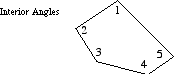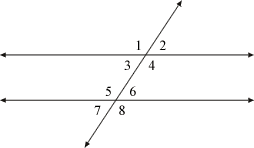index: click on a letter A B C D E F G H I J K L M N O P Q R S T U V W X Y Z A to Z index index: subject areas numbers & symbols sets, logic, proofs geometry algebra trigonometry advanced algebra & pre-calculus calculus advanced topics probability & statistics real world applications multimedia entrieswww.mathwords.com about mathwords website feedback

Interior Angle

An angle on the interior of a plane figure.

Examples: The angles labeled 1, 2, 3, 4, and 5 in the pentagon below are all interior angles. Angles 3, 4, 5, and 6 in the second example below are all interior angles as well (parallel lines cut by a transversal).

Note: The sum of the interior angles of an n-gon is given by the formula (n – 2)·180°. For a triangle this sum is 180°, a quadrilateral 360°, a pentagon 540°, etc.Parallel lines cut by a transversal